Properties of An Isosceles Triangle - Triangle, Class 9, Mathematics

# Properties of An Isosceles Triangle - Triangle, Class 9, Mathematics - Extra Documents & Tests for Class 9

PROPERTIES OF AN ISOSCELES TRIANGLE

In this section, we will learn some properties related to a triangle whose two sides are equal. We know that a triangle whose two sides are equal is called an isosceles triangle.
Here, we will apply SAS congruence criteria and ASA (or AAS) congruence criteria to study some properties of an isosceles triangle.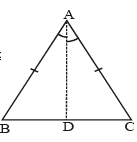THEOREM-3 : Angles opposite to equal sides of an isosceles triangle are equal.
Given : ΔABC is an isosceles triangle and AB = AC.
To prove : ∠B =  ∠C.
Construction : Draw AD the bisector of ∠A. AD meets BC at D.
Proof :

Hence, proved.

COROLLARY : Each angle of an equilateral triangle is of 60°.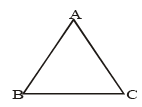Given : ΔABC is an equilateral triangle.
To prove : ∠A = ∠B = ∠C = 60°.
Proof :

 STATEMENT REASON 1. AB = AC = BC ΔABC is an equilateral triangle. 2. ∠B = ∠C AB = AC 3. ∠A = ∠C AB = BC 4. ∠A = ∠B = ∠C From (2) and (3) 5. ∠A + ∠B + ∠C = 180°⇒ ∠A=∠B=∠C= 1/3 × 180°=60° Angle sum property.

Hence, proved.

THEOREM-4 : The sides opposite to equal angles of a triangle are equal.
Given : ΔABC in which ∠B = ∠C.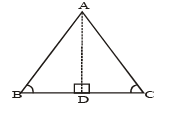To prove : AB = AC.
Proof :

 STATEMENT REASON 1. In ΔABD and ΔACD(i) ∠ABD = ∠ACD(ii) ∠ADB = ∠ADC(iii) AD = AD GivenEach = 90°Common 2. ΔABD ≌ ΔACD By AAS criteria 3. AB = A C C.P.C.T.

Ex.16 In the adjoining fig, find the value of x.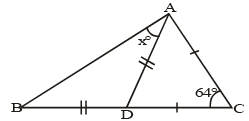But CD = CA  ⇒ ∠CAD = ∠ADC [∠s opposite to equal sides of a D are equal]
So, ∠CAD = ∠ADC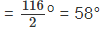Now, ∠AD C = ∠AB D + ∠DA B [Ext. ∠ of a D = sum of int. opp. ∠s]
But, AD = BD  ⇒ ∠ABD = ∠DAB.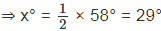Hence, x = 29.

Ex.17 ΔABC is an isosceles triangle in which AB = AC. Side BA is produced to D such that AD = AB.
Show that ∠BCD is a right angle.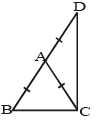Sol. Given : ΔABC is an isosceles triangle in which AB = AC.
Side BA is produced to D such that AD = AB

To prove : ∠BCD = 90°

Proof :

 STATEMENT REASON 1. AB = A C   ⇒ ∠ABC = ∠ACB Given∠s opposite to equal sides of a D are equal 2. A B = AD Given 3. AC = A D ⇒ ∠CD A = ∠AC Dor ∠CDB = ∠ACD From (1) & (2)∠s opposite to equal sides of a D are equal 4. ∠A B C + ∠C D B = ∠A C B + ∠A C D ⇒ ∠AB C + ∠CD B = ∠BC D Adding (1) & (3) 5. In ΔBCD ∠BCD + ∠DBC + ∠CDB = 180° ⇒ ∠BCD + ∠ABC + ∠CDB = 180° ⇒ ∠BCD + ∠BCD = 180° ⇒ 2∠BCD = 180° ⇒ ∠BCD = 90° ⇒ ∠BCD is a right angle. Angle sum propertyUsing (4)

Hence, proved.

Ex.18 In a right angled triangle, one acute angle is double the other.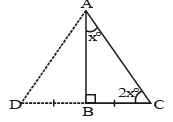Prove that the hypotenuse is double the smallest side.
Sol. Given :  ΔABC in which ∠B = 90° and ∠ACB = 2 ∠CAB.
To prove : AC = 2BC.
Construction : Produce CB to D such that BD = BC. Join AD.
Proof :

 STATEMENT REASON 1. In ΔABD and ΔABC(i) BD = BC(ii) ∠ABD = ∠ABC(iii) AB = AB By construction.Each = 90°Common 2. ΔABD ≌  ΔA B C By SAS criteria 3. AD = AC, ∠DAB = ∠CAB = x° ⇒ ∠DA C = ∠AC B C.P.C.T 4. A D = D C Sides opposite to equal ∠s are equal. 5. A C = D C AD = AC, from (3) 6. AC = 2 BC DC = BD + BC = 2BC, as BD = BC.

Hence, proved.

INEQUALITIES IN A TRIANGLE

THEOREM-5 : If two sides of a triangle are unequal, then the greater side has greater angle opposite to it.
Given :
ΔABC in which AC > AB.
To prove : ∠ABC > ∠ACB.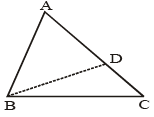Construction : Mark a point D on AC such that AD = AB. Join BD.
Proof : ST

 STATEMENT REASON 1. A B = A D By construction 2. ∠A B D = ∠B D A ∠s opposite to equal sides of a D are equal 3. ∠B D A > ∠D C B (Ext. ∠ of ΔBCD) > (Each of its int. Opp. ∠s) 4. ∠A B D > ∠D C B Using (2) 5. ∠AB C > ∠AB D ∠ABD is a part of ∠ABC. 6. ∠AB C > ∠DC B Using (5) 7. ∠ABC > ∠AC B ∠DCB = ∠ACB.

THEOREM-6 (Converse of Theorem-5) : If two angles of a triangle are unequal, then the greater angle has greater side opposite to it.
Given :
ΔABC in which ∠ABC > ∠ACB.
To prove : AC > AB.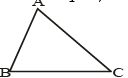Proof :

 STATEMENT REASON We may have three possibilities only : (i) AC = AB(ii) AC < AB(iii) AC > ABOut of these exactly one must be true. AC = AB. Case-I.  ⇒ ∠ABC = ∠ACB.This is contrary to what is given.∴ AC = AB is not true. ∠s opposite to equal sides of a D are equal Case-II. AC < AB.  ⇒ AB > AC ⇒ ∠ACB > ∠ABCThis is contrary to what is given. ∴ AC < AB is not true.Thus, we are left with the only possibility AC > AB, which must be true. Greater side has greater angle opp. to it.

Hence, proved.

THEOREM-7 : The sum of any two sides of a triangle is greater than its third side.
Given :
ΔABC.
To prove :
(i)  AB + AC > BC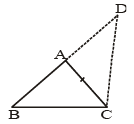(ii) AB + BC > AC (iii) BC + AC > AB.
Construction : Produce BA to D such that AD = AC. Join CD.
Proof :

 STATEMENT REASON 1. A D = A C ⇒ ∠AC D = ∠AD C By construction 2. ∠B C D > ∠A C D ∠s opposite to equal sides of a D are equal 3. ∠B C D > ∠A D C ⇒ BD > B C ⇒ BA + AD > BC ⇒ BA + AC > BCor AB + AC > BC. Using (1) & (2)Greater angle has greater side opp. to it.BAD is a straight line, BD = BA + AD. 4. Similarly, AB + BC > AC and BC + AC > AB. AD = AC, by construction

REMARK : (i) The largest side of a triangle has the greatest angle opposite to it and converse is also true. (ii) The smallest side of a triangle has the smallest angle opposite to it and converse in also true.

Ex.19 In fig, show that : (i) AB > AC (ii) AB > BC and (iii) BC > AC.
Sol. Given : A ΔABC in which ∠B = 40° and ∠ACD = 100°.
To prove : (i)  AB > AC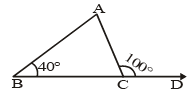(ii) AB > BC (iii) BC > AC.
Proof :

 STATEMENT REASON 1. ∠A + ∠B = 100° ⇒ ∠A + 40° = 100° ⇒ ∠A = 60° Ext. ∠ = sum of int. opt. ∠sLinear pair of angles. 2. ∠C + 100° = 180° ⇒ ∠C = 80° ∠C = 80° and ∠B = 40°Greater angle has greater side opp. to it. 3. ∠C > ∠B ⇒ AB > AC ∠C = 80° and ∠A = 60°Greater angle has greater side opp. to it. 4. ∠C > ∠A ⇒ AB > BC ∠A = 60° and ∠B = 40° 5. ∠A > ∠B ⇒ BC > AC Greater angle has greater side opp. to it.

Hence, proved.

Ex.20 Show that of all the line segments that can be drawn to a given straight line from a given point outside it, the perpendicular is the shortest.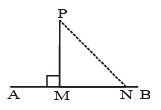Sol. Given : A straight line AB and a point P outside it; PM ⊥ AB and N is any other point on AB.
To prove : PM < PN.
Proof :

 STATEMENT REASON 1. ∠PMN = 90° P M ⊥ A B 2. ∠PNM < 90° In a right D, one angle measures 90° and each one of the remaining two is acute. 3. ∠P N M < ∠P M N From (1) and (2) 4. P M < P N Side opp. the smaller angle is smaller.

 THINGS TO REMEMBER1. Two figures are congruent, if they are of the same shape and of the same size.2. Two circles of the same radii are congruent.3. Two squares of the same sides are congruent.4. If two triangles ABC and PQR are congruent under the correspondence A ⇔  P, B ⇔  Q and C ⇔  R, then symbolically, it is expressed as Δ ABC ≌ Δ PQR.5. If two sides and the included angle of one triangle are equal to two sides and the included angle of the other triangle, then the two triangles are congruent (SAS congruence rule).6. If two angles and the included side of one triangle are equal to two angles and the included side of the other triangle, then the two triangles are congruent (ASA congruence rule).7. If two angles and one side of one triangle are equal to two angles and the corresponding side of the other triangle, then the two triangles are congruent (AAS Congruence rule).8. Angle opposite to equal sides of a triangle are equal.9. Sides opposite to equal angles of a triangle are equal.10. Each angle of an equilateral triangle is of 60°.11. If three sides of one triangle are equal to three sides of the other triangle, then the two triangles are congruent (SSS congruence rule).12. If in two right triangles, hypotenuse and one side of a triangle are equal to the hypotenuse and one side of other triangle, then the two triangles are congruent (RHS congruence rule).13. In a triangle, angle opposite to the longer side is larger (greater).14. In a triangle, side opposite to the larger (greater) angle is longer.15. Sum of any two sides of a triangle is greater than the third side.
The document Properties of An Isosceles Triangle - Triangle, Class 9, Mathematics | Extra Documents & Tests for Class 9 is a part of the Class 9 Course Extra Documents & Tests for Class 9.
All you need of Class 9 at this link: Class 9

## Extra Documents & Tests for Class 9

1 videos|228 docs|21 tests

## FAQs on Properties of An Isosceles Triangle - Triangle, Class 9, Mathematics - Extra Documents & Tests for Class 9

 1. What is an isosceles triangle?Ans. An isosceles triangle is a type of triangle that has two sides of equal length. The angles opposite to the equal sides are also equal in an isosceles triangle.
 2. What are the properties of an isosceles triangle?Ans. The properties of an isosceles triangle are as follows: - It has two sides of equal length. - The angles opposite to the equal sides are also equal. - The base angles (angles adjacent to the base) are equal. - The base (the side opposite to the vertex angle) is the longest side in the triangle. - The altitude from the vertex angle bisects the base and is also the perpendicular bisector of the base.
 3. Can an isosceles triangle have all angles equal?Ans. No, an isosceles triangle cannot have all angles equal. It can only have two angles equal, which are the angles opposite to the equal sides. The third angle, known as the vertex angle, can be different from the other two angles.
 4. How can we find the measure of the angles in an isosceles triangle?Ans. In an isosceles triangle, if we know the measure of one angle, we can find the measure of the other two angles. Since the base angles are equal, we can subtract the given angle from 180 degrees and then divide the result by 2 to find the measure of each base angle. The vertex angle can be found by subtracting the sum of the base angles from 180 degrees.
 5. Are all equilateral triangles isosceles?Ans. Yes, all equilateral triangles are isosceles. An equilateral triangle is a special type of isosceles triangle in which all three sides and angles are equal. Therefore, it satisfies the properties of an isosceles triangle as well.

## Extra Documents & Tests for Class 9

1 videos|228 docs|21 testsExplore Courses for Class 9 examSignup to see your scores go up within 7 days! Learn & Practice with 1000+ FREE Notes, Videos & Tests.
10M+ students study on EduRev
Track your progress, build streaks, highlight & save important lessons and more!
Related Searches

,

,

,

,

,

,

,

,

,

,

,

,

,

,

,

,

,

,

,

,

,

,

,

,

,

,

,

;